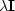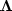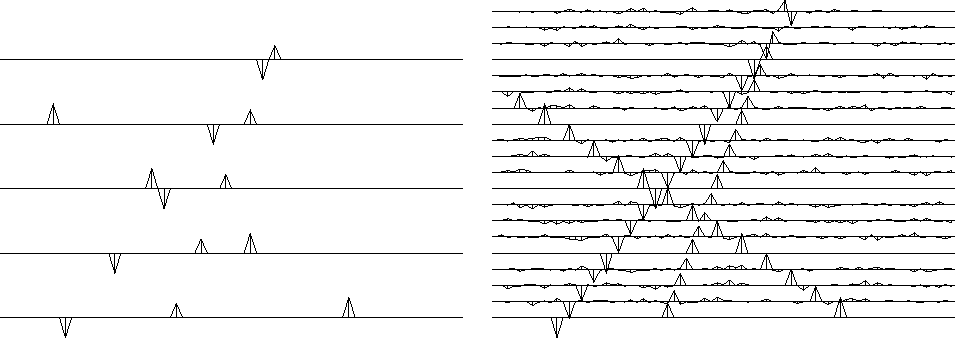Next: Narrow-band data Up: 2-D INTERPOLATION BEYOND ALIASING Previous: The regression codes

## Zapping the null space with envelope scaling

Here we will see how to remove the small noise we are seeing in the interpolated outputs. The filter (10) obviously destroys the input in Figure 14. On the output interpolated data, the filter-output residuals (not shown) were all zeros despite the small noises. The filter totally extinguishes the small noise on the outputs because the noise has the same stepout (slope) as the signals. The noise is absent from the original traces, which are interlaced. How can dipping noises exist on the interpolated traces but be absent from the interlaced data? The reason is that one dip can interfere with another to cancel on the known, noise-free traces. The filter (10) destroys perfect output data as well as the noisy data in Figure 14. Thus, there is more than one solution to the problem. This is the case in linear equation solving whenever there is a null space. Since we manufactured many more data points than we originally had, we should not be surprised by the appearance of a null space. When only a single dip is present, the null space should vanish because the dip vanishes on the known traces, having no other dips to interfere with it there. Confirm this by looking back at Figure 17, which contains no null-space noise. This is good news, because in real life, in any small window of seismic data, a single-dip model is often a good model.

If we are to eliminate the null-space noises, we will need some criterion in addition to stepout. One such criterion is amplitude: the noise events are the small ones. Before using a nonlinear method, we should be sure, however, that we have exploited the full power of linear methods. Information in the data is carried by the envelope functions, and these envelopes have not been included in the analysis so far. The envelopes can be used to make weighting functions. These weights are not weights on residuals, as in the routine iner(). These are weights on the solution. Thestabilization in routine pe2()applied uniform weights using the subroutine ident(), as has been explained. Here we simply apply variable weightsusing the subroutine diag(). The weights themselves are the inverse of the envelope of input data (or the output of a previous iteration). Where the envelope is small lies a familiar problem, which I approached in a familiar way--by adding a small constant. The result is shown in Figure 19.wlace3
Figure 19
Top left is input. Top right is the interpolation with uniform weights. In the middle row are the envelope based on input data and the corresponding interpolated data. For the bottom row, the middle-row solution was used to design weights from which a near-perfect solution was derived.

The top row is the same as Figure 13. The middle row shows the improvement that can be expected from weighting functions based on the inputs. So the middle row is the solution to a linear interpolation problem. Examining the envelope function on the middle left, we can see that it is a poor approximation to the envelope of the output data, but that is to be expected because it was estimated by smoothing the absolute values of the input data (with zeros on the unknown traces). The bottom row is a second stage of the process just described, where the new weighting function is based on the result in the middle row. Thus the bottom row is a nonlinear operation on the data.

When interpolating data, the number of unknowns is large. Here each row of data is 75 points, and there are 20 rows of missing data. So, theoretically, 1500 iterations might be required. I was getting good results with 15 conjugate-gradient iterations until I introduced weighting functions; then the required number of iterations jumped to about a hundred. The calculation takes seconds (unless the silly computer starts to underflow; then it takes me 20 times longer.)

I believe the size of the dynamic range in the weighting function has a controlling influence on the number of iterations. Before I made Figure 19, I got effectively the same result, and more quickly, using another method, which I abandoned because its philosophical foundation was crude. I describe this other method here only to keep alive the prospect of exploring the issue of the speed of convergence. First I moved the do iter'' line above the already indented lines to allow for the nonlinearity of the method. After running some iterations withto ensure the emergence of some big interpolated values, I turned onat values below a threshold. In the problem at hand, convergence speed is not important economically but is of interest because we have so little guidance as to how we can alter problem formulation in general to increase the speed of convergence.Next: Narrow-band data Up: 2-D INTERPOLATION BEYOND ALIASING Previous: The regression codes
Stanford Exploration Project
10/21/1998